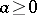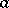# Arithmetic root

(diff) ← Older revision | Latest revision (diff) | Newer revision → (diff)
Jump to: navigation, search

arithmetical value of the-th root of a real numberA non-negative number the-th power of which is equal to. In considering the two real values of the-th root, whereis an even number, of a non-negative number, one speaks of the algebraic values of the root in the domain of the real numbers; if, on the other hand, one is considering allvalues of an-th root, then one speaks of the values of the root in the domain of the complex numbers.

How to Cite This Entry:
Arithmetic root. Encyclopedia of Mathematics. URL: http://encyclopediaofmath.org/index.php?title=Arithmetic_root&oldid=19182